﻿ Transient AnalysisExamples | Product | Murata Software Co., Ltd.# Example4Transient Analysis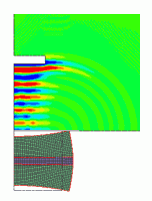### General

• This is a transient analysis of piezoelectric-acoustic coupled analysis.

• A piezoelectric material is driven by voltage to generate sound wave propagation.
• Unless specified in the list below, the default conditions will be applied.

### Analysis Condition

 Item Setting Solver Piezoelectric analysis [Rayleigh] Acoustic analysis [Mach] Analysis Space 2D Analysis Type Piezoelectric analysis: transient-resonant analysis Acoustic analysis: transient analysis Unit mm

Tab

Setting Item

Setting

Mesh

Meshing Setup

Element type: rectangle

☑ Create square meshes

Resonant Analysis

Resonant Analysis

Number of modes: 3

Approximated frequency: 0

Transient Analysis

Timestep

Manual

 No Calculation steps Output interval Timestep [s] 1 250 1 2.0e-6

Solution Method (Acoustic Analysis)

Implicit method

• The timestep is determined based on the resonant frequency and the applied frequency. The resonance at 41kHz is confirmed by a resonant analysis performed in advance. Therefore the applied frequency is set at 40kHz, which is slightly lower than the resonance.
At 40kHz, the period T0 is T0=1/40e3=2.5e-5. Timestep (dT) is required to be or less than 1/10 of the period. So the value is set to dT=2.0e-6[s], which is slightly smaller than 1/10 of the period.
Finish time of calculation is 250×2.0e-6=5e-4[s]=500[us]. A driving frequency is required when setting the electric potential-specified boundary condition.

### Model

The model is a half model in 2D.

The domain for the piezoelectric analysis consists of an piezoelectric material and stainless steel. The electric potential-specified boundary conditions of hot and earth are set to the piezoelectric material. The electric potential 0[V] is specified for “earth” and the voltage oscillating in sine wave is specified for “hot”.

On the edge of X=0, a boundary condition with X displacement of zero is set.

The domain for acoustic analysis is air. The open boundary condition is set to the domain for the acoustic analysis.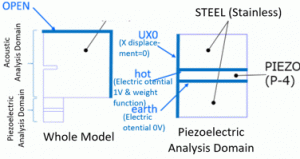### Body Attributes and Materials

 Body Number/Type Body Attribute Name Material Name 0/Sheet STEEL 104_Stainless_steel * 1/Sheet PIEZO 000_P4 * 2/Sheet STEEL 104_Stainless_steel * 13/Sheet AIR 000_Air *

(*) Available from the material DB. However, the value of 1/Qm for 000_P4 is changed to 0.4 in order to suppress the vibration after driving by voltage.

 Body Attribute Name Analysis Domain (Solver) PIEZO Piezoelectric Analysis (Rayleigh) STEEL Piezoelectric Analysis (Rayleigh) AIR Acoustic Analysis (Mach)

• Cautions: In this analysis, it is necessary to specify the analysis domain. Specify either piezoelectric analysis or acoustic analysis on the analysis domain tab.

### Boundary Condition

 Boundary Condition Name/Topology Tab Boundary Condition Type Setting OPEN/Edge Acoustic Open boundary US0/Edge Mechanical Displacement UX=0, UY=0 hot/Edge Electric Electric potential Specify electric potential = 1[V] Select “Time dependency” Weight is as in the graph below earth/Edge Electric Electric potential Specify voltage = 0[V]

The driving frequency is set to 40kHz as explained in the analysis condition. Using a spreadsheet, the sine wave of 40kHz is created, and part of it is used for the graph of [time – weight].

The numerical data created on the spreadsheet can be copied and pasted to the [Time – Weight] Table.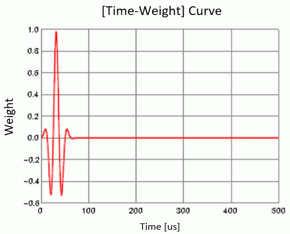### Results

##### 1. Piezoelectric Analysis
 Piezoelectric Analysis 1: 4.107499e+04Hz Displacement [m] Z component 0° Linear

The result fields can be viewed.

At [Results] tab – [Display] – [Analysis type], select “piezoelectric analysis” to see the results of the piezoelectric-resonant analysis.

Of the three vibration modes that were solved, this mode has the largest coupling coefficient. The coupling coefficient can be confirmed in the result table.

The figure below is a contour diagram of Z displacement drawn over the displacement diagram.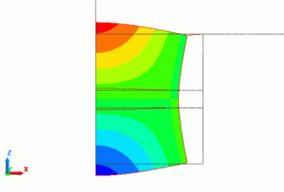Fig. 1

##### 2. Piezoelectric/Time response
 Piezoelectric/Time response 24: 4.800000e-05[s] Displacement [m] Z component Linear

At [Results] tab – [Display] – [Analysis type], select “piezoelectric/time response” to see the results of the transient-resonant analysis.

Here, you can examined the vibration when the [time – weight] curve is applied. The [time- weight] curve was specified for the boundary condition “hot”. Fig. 2(b) is a Z displacement graph that is observed at a point A in Fig. 2(a). The mode is on the horizontal axis.

Fig. 2(a) is a contour diagram at the time indicated by a marker in Fig. 2(b). A contour diagram is drawn over the displacement diagram. The vibration occurs in the mode shown in Fig. 1.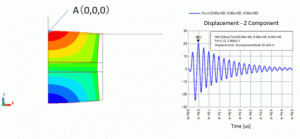Fig. 2(a) Fig. 2(b)

##### 3. Acoustic Analysis
 Acoustic Analysis 82: 1.640000e-04[s] Sound pressure [Pa] Value Linear

At [Results] tab – [Display] – [Analysis type], select “acoustic analysis” to see the results of the acoustic analysis.

The sound pressure distribution is shown in Fig. 3. The sound pressure driven by the piezoelectric material reaches the obstacle placed in the center.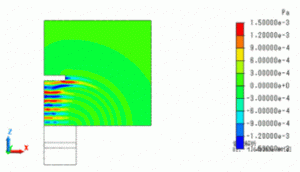Fig. 3

Fig. 4 is a power graph. In the Result Table, set analysis type to [Acoustic Analysis], set selection mode to [Show all results summary] , and select [Power] tab. Then press Graph button to draw a graph with time on the horizontal axis and power on the vertical axis.

The power becomes negative at around 300us. It indicates that the waves return after reflection at the obstacle.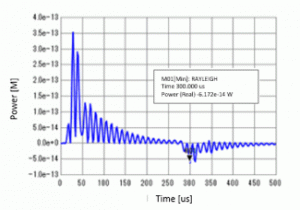Fig. 4

The sound pressure distribution at 300[us] is shown below. As expected, it is confirmed that the reflected waves return to the piezoelectric material from the obstacle.

 Acoustic Analysis 150: 3.0000e-04[s] Sound pressure [Pa] Value Linear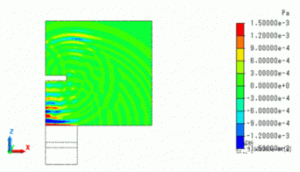Fig. 5

﻿